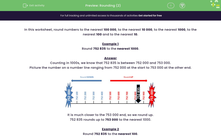# Rounding (2)

In this worksheet, students round any number up to 1 000 000 to the nearest 10, 100, 1000, 10 000 or 100 000.Key stage:  KS 2

Curriculum topic:   Number: Number and Place Value

Curriculum subtopic:   Round Numbers to 1 000 000

Difficulty level:#### Worksheet Overview

In this worksheet, round numbers to the nearest 100 000, to the nearest 10 000, to the nearest 1000, to the nearest 100 and to the nearest 10.

Example 1

Round 752 835 to the nearest 1000.

Counting in 1000s, we know that 752 835 is between 752 000 and 753 000.

Picture the number on a number line ranging from 752 000 at the start to 753 000 at the other end.It is much closer to the 753 000 end, so we round up.

752 835 rounds up to 753 000 to the nearest 1000.

Example 2

Round 752 835 to the nearest 100.

Counting in 100s, we know that 752 835 is between 752 800 and 752 900.

Picture the number on a number line ranging from 752 800 at the start to 752 900 at the other end.It is closer to the 752 800 end, so we round down.

752 835 rounds down to 752 800 to the nearest 100.

Example 3

Round 752 835 to the nearest 10.

Counting in 10s, we know that 752 835 is between 752 830 and 752 840.

Picture the number on a number line ranging from 752 830 at the start to 752 840 at the other end.It is exactly in the middle, so we round up

Remember that we round up when it is halfway.

752 835 rounds up to 752 840 to the nearest 10.

### What is EdPlace?

We're your National Curriculum aligned online education content provider helping each child succeed in English, maths and science from year 1 to GCSE. With an EdPlace account you’ll be able to track and measure progress, helping each child achieve their best. We build confidence and attainment by personalising each child’s learning at a level that suits them.

Get started